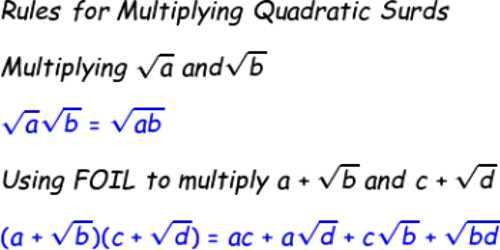Definitions of surds: A root of a positive real quantity is called a surd if its value cannot be exactly determined. It is a number that can’t be simplified to remove a square root (or cube root etc). For example, each of the quantities √3, ∛7, ∜19, (16)^2/5 etc. is a surd.

More Examples:

• √2 (square root of 2) can’t be simplified further so it is a surd
• √4 (square root of 4) CAN be simplified to 2, so it is NOT a surd

A quadratic surd is an irrational number that is the solution to some quadratic equation with rational coefficients which is irreducible over the set of rational numbers. A number of the form (±√a), where ‘a’ is a positive rational number which is not the square of another rational number is called a pure quadratic surd. A number of the form (a ± √b), where ‘a’ is rational √b is a pure quadratic surd is sometimes called a mixed quadratic surd.Product of two unlike Quadratic Surds

The product of two, unlike quadratic surds, cannot be rational.

Suppose, let √p and √q be two, unlike quadratic surds.

We have to show that √p ∙ √q cannot be rational.

If possible, let us assume, √p ∙ √q = r where r is rational.

Therefore, √q = r/√p = (r ∙ √p)/(√p ∙ √p) =  (r /p) √p

√q = (a rational quantity) √p, [Since, r and p both are rational, therefore, r/p is rational.)

Now from the above expression we clearly see that √p and √q are like surds, which is a contradiction. Therefore, our assumption cannot hold i.e., √p ∙ √q cannot be rational.

Therefore, the product of two, unlike quadratic surds, cannot be rational.

Notes:

1. In like manner, we can show that the quotient of two, unlike quadratic surds, cannot be rational.
2. The product of two like quadratic surds always represents a rational quantity.

For example, consider two like quadratic surds m√z and n√z where m and n are rational.

Now the product of m√z and n√z = m√z ∙ n√z = mn(√z^2)= mnz, which is a rational quantity.

1. The quotient of two like quadratic surds always represents a rational quantity. For example, consider, For example, consider two like quadratic surds m√z and n√z where m and n are rational.

Now the quotient of m√z and n√z = (m√z)/(n√z) = m/n, which is a rational quantity.

Information Source: